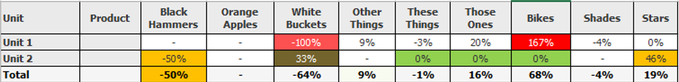# QlikView App Dev

Discussion Board for collaboration related to QlikView App Development.

Announcements
Now Live: Qlik Sense SaaS Simplified Authoring – Analytics Creation for Everyone: READ DETAILS
cancel
Showing results for
Did you mean:Creator II

HI,

I have a relatively simple Pivot table with two Dimensions : Unit (down the left) and Product as Column Headings.

My expression is a simple percentage :  (sum(ordered)  - sum(provided )) / sum(provided)

One thing to note is that there are some negative percentages (which is expected).  What I would like to do is Color the expression values to something like the following :Is it possible to colorize the expression as Red for the highest values (eg 100% and 167% as above moving towards green to 0%) with amber for the middle values.

Im trying to show the movement away from 0% for both negative and positive values.  Also, would colormix merge the colors into a gradient?

Phil

1 Solution

Accepted SolutionsMVP

@prees959  try below expression in background color expression

``````=ColorMix1 (fabs((sum(ordered)  - sum(provided )) / sum(provided))
/
\$(=max(aggr(fabs((sum(ordered)  - sum(provided )) / sum(provided)),Unit,Product))),
ARGB(255, 255, 0, 0), ARGB(255, 0, 255, 0))``````
3 RepliesMVP

@prees959  try below expression in background color expression

``````=ColorMix1 (fabs((sum(ordered)  - sum(provided )) / sum(provided))
/
\$(=max(aggr(fabs((sum(ordered)  - sum(provided )) / sum(provided)),Unit,Product))),
ARGB(255, 255, 0, 0), ARGB(255, 0, 255, 0))``````Creator II
Author

hi @Kushal_Chawda  - thanks so much for your reply.  It seems to work but for some reason its missing out some of the higher values :any ideas?

ThanksMVP

@prees959  or check the colormix wizard in background expression.  Put below expression for colormix wizard and then you can set the upper, lower and intermediate settings and set the colors

`fabs((sum(ordered)  - sum(provided )) / sum(provided))`